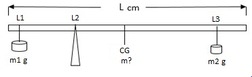# Problem 55550. Find the mass of a rod

Determine the mass (in grams) of a rod of length L cm if it is in equilibrium per the diagram. In the diagram L1, L2, L3 are distance from the left end of the rod, in the order specified (i.e, L3>L2>L1). Assume the center of gravity is at the midpoint of the rod. Find the mass to the nearest gram.### Solution Stats

55.56% Correct | 44.44% Incorrect
Last Solution submitted on Dec 09, 2023

### Community Treasure Hunt

Find the treasures in MATLAB Central and discover how the community can help you!

Start Hunting!